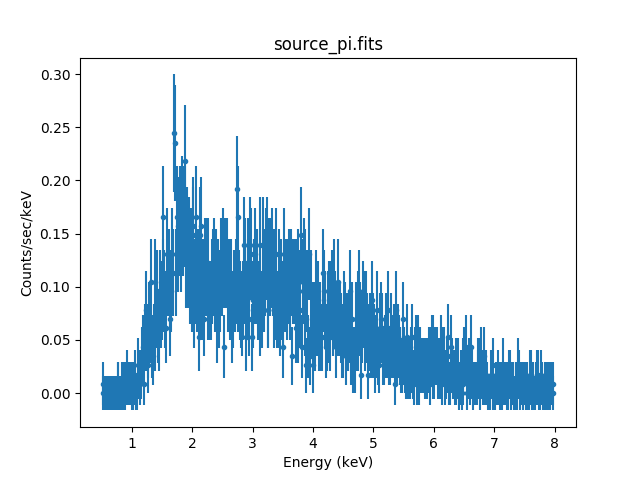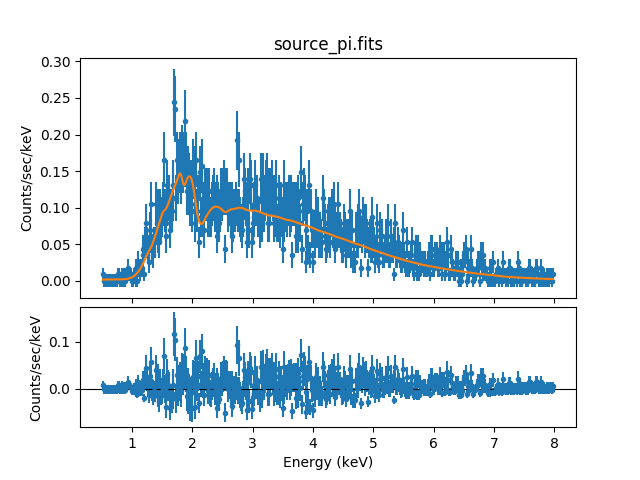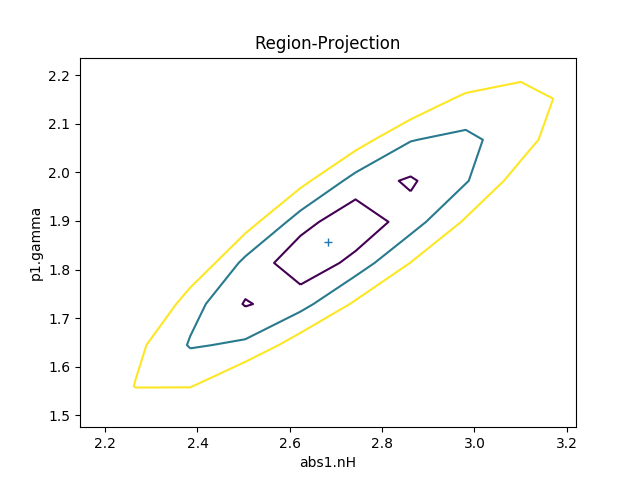# Fitting PHA Data with Multi-Component Source ModelsSherpa Threads (CIAO 4.11 Sherpa v1)

## Overview

#### Synopsis:

This thread shows how to do a basic spectral fit with the appropriate response files. If you are interested in including the background in the fit as well, see the Simultaneously Fitting Source and Background Spectra thread.

Last Update: 10 Dec 2018 - updated for CIAO 4.11, screen output revised

## Reading Data & Instrument Responses Into Sherpa

In this thread, we wish to fit spectral data from the FITS PHA data file source_pi.fits. This data set is input to Sherpa with the load_pha function:

```sherpa> load_pha("source_pi.fits")
statistical errors were found in file 'source_pi.fits'
but not used; to use them, re-read with use_errors=True
```

The instrument response for a data set is established when the appropriate response files (ARF, RMF) are loaded into the Sherpa session. If the instrument response files are written in the header of the PHA file, Sherpa will load them automatically; if not, as in this example, they need to be loaded manually with the load_arf and load_rmf commands.

```sherpa> load_arf("arf.fits")
```

The current definition of the instrument response may be examined using show_all:

```sherpa> show_all()
Data Set: 1
Filter: 0.0080-14.9431 Energy (keV)
Noticed Channels: 1-1024
name           = source_pi.fits
channel        = Float64
counts         = Float64
staterror      = None
syserror       = None
bin_lo         = None
bin_hi         = None
grouping       = None
quality        = None
exposure       = 7854.46647487
backscal       = 2.36640571175e-06
areascal       = 1.0
grouped        = False
subtracted     = False
units          = energy
rate           = True
plot_fac       = 0
response_ids   = 
background_ids = []

RMF Data Set: 1:1
name     = rmf.fits
detchans = 1024
energ_lo = Float64
energ_hi = Float64
n_grp    = UInt64
f_chan   = UInt32
n_chan   = UInt32
matrix   = Float64
offset   = 1
e_min    = Float64
e_max    = Float64
ethresh  = 1e-10

ARF Data Set: 1:1
name     = arf.fits
energ_lo = Float64
energ_hi = Float64
specresp = Float64
bin_lo   = None
bin_hi   = None
exposure = 7854.46689558
ethresh  = 1e-10
```

This output shows that rmf.fits and arf.fits currently define the instrument response.

## Filtering Spectral Data

Sherpa has many filtering options with the ignore and notice functions. During this Sherpa session, we would like to fit only the data between 0.5 and 8.0 keV. To apply this filter to the data set, we use ignore:

```sherpa> ignore(":0.5, 8.0:")
```Analysis Units

Note that the units of the arguments supplied to the ignore / notice functions must match the units of the data set. The data units can be changed with the get_data or set_analysis functions:

```sherpa> set_analysis("energy")

OR

sherpa> get_data().units="energy"

sherpa> print(get_data().units)
energy
```

To remove this filter:

```sherpa> notice()
```

The filter may then be re-applied (or a different filter may be applied):

```sherpa> ignore(":0.5, 8.0:")
```

The filtered data set may then be plotted:

```sherpa> plot_data()
```

Figure 1 shows the resulting plot. Notice that the plot now includes only the data in the specified energy region.

### Figure 1: Source spectrum (0.5-8.0 keV)## Defining a Multi-Component Source Model Expression

We will fit the spectral data using a source model expression that involves multiple model components.

The first of these model components is the one-dimensional power-law model 'powlaw1d'. The second of the model components is an XSpec photoelectric absorption model called 'xsphabs' in Sherpa. Please see the XSpec User's Guide for more information about this model. Note that all XSpec models are available from within Sherpa and their XSpec names are preceded by xs.

We define an expression that is the product of the two model components. Below, the 'powlaw1d' and 'xsphabs' model components are named p1 and abs1, respectively, and the multi-component source model for fitting the data is defined with the set_source function:

```sherpa> set_source(xsphabs.abs1*powlaw1d.p1)
```

We set the initial value of the equivalent neutral hydrogen column density of model abs1 to 1e-07 (in units of 1022 atoms/cm2):

```sherpa> abs1.nH = 1e-07
```

The guess function may be used to estimate initial parameter values for a model, as well as the minima and maxima for their ranges; where guess() is not able to estimate initial parameter values, we set our own. To have Sherpa automatically query for the initial parameter values when a model is established, set 'paramprompt(True)' (it is 'False' by default).

The current definition of the Sherpa source model expression, including initial parameter values for each model component, may be examined using show_model:

```sherpa> show_model()
Model: 1
apply_rmf(apply_arf((7854.46647487 * (xsphabs.abs1 * powlaw1d.p1))))
Param        Type          Value          Min          Max      Units
-----        ----          -----          ---          ---      -----
abs1.nH      thawed        1e-07            0       100000 10^22 atoms / cm^2
p1.gamma     thawed            1          -10           10
p1.ref       frozen            1 -3.40282e+38  3.40282e+38
p1.ampl      thawed            1            0  3.40282e+38

sherpa> print(abs1.integrate)
True

sherpa> print(p1.integrate)
True
```

This output shows that abs * p1 is currently defined as the source model expression. By default, integration over an energy bin is turned on for both the abs1 and p1 model components; a change to the integration flag will not change the fit. Since we are using a multiplicative source model expression to fit binned PHA data, integration of the source expression over each energy bin will be performed during fitting.

As of the release of Sherpa v2 in CIAO 4.2, we may choose to explicitly set the complete convolved model expression to be used for fitting a source data set, using the new function set_full_model, together with get_response or get_arf/get_rmf (an associated background spectrum may be fit simultaneously using set_bkg_full_model; see the threads Simultaneously Fitting Source and Background Spectra and Fitting a PHA Data Set with Multiple Responses for examples). The set_full_model function offers an alternative to using set_source, which automatically convolves the appropriate instrument response with the defined source model expression—but which does not allow for applying separate responses to individual model components within a single model expression, e.g., to leave some model components unconvolved by the instrument response.

The following set of commands represents the manual definition of the chosen source model, which was automatically (and equivalently) defined above with set_source.

```sherpa> rsp = get_response()
sherpa> set_full_model(rsp(xsphabs.abs1*powlaw1d.p1))
```

The get_response function returns the ARF×RMF instrument response model which can be used to explicitly convolve the source model. Multiplication by the source exposure time is done implicitly.

We could also use get_arf and get_rmf in place of get_response, as follows:

```sherpa> arf = get_arf()
sherpa> rmf = get_rmf()

sherpa> set_full_model(rmf(arf(xsphabs.abs1*powlaw1d.p1)))
```

It is important to note that the Sherpa functions which are related to a source or background model defined with set_source or set_bkg_source—such as plot_source/plot_bkg_source or calc_energy_flux—are not compatible with the complete model expression defined by the set_full_model or set_bkg_full_model functions. In order to use these Sherpa functions, users should define source and background models in the usual way with the automatic functions set_source and set_bkg_source.)

## Modifying Method & Statistic Settings

The show_method and show_stat functions may be used to view the current method and statistics settings:

```sherpa> show_method()
Optimization Method: LevMar
name    = levmar
ftol    = 1.19209289551e-07
xtol    = 1.19209289551e-07
gtol    = 1.19209289551e-07
maxfev  = None
epsfcn  = 1.19209289551e-07
factor  = 100.0
verbose = 0

sherpa> show_stat()
Statistic: Chi2Gehrels
Chi Squared with Gehrels variance.

The variance is estimated from the number of counts in each bin,
but unlike `Chi2DataVar`, the Gaussian approximation is not
used. This makes it more-suitable for use with low-count data.

The standard deviation for each bin is calculated using the
approximation from _:

sigma(i,S) = 1 + sqrt(N(i,s) + 0.75)

where the higher-order terms have been dropped. This is accurate
to approximately one percent. For data where the background has
not been subtracted then the error term is:

sigma(i) = sigma(i,S)

whereas with background subtraction,

sigma(i)^2 = sigma(i,S)^2 + [A(S)/A(B)]^2 sigma(i,B)^2

Notes
-----
The accuracy of the error term when the background has been
subtracted has not been determined. A preferable approach to
background subtraction is to model the background as well as the
source signal.

References
----------

..  "Confidence limits for small numbers of events in
astrophysical data", Gehrels, N. 1986, ApJ, vol 303,
p. 336-346.
```

We will change the statistic to Chi2XSpecVar for fitting this data:

```sherpa> set_stat("chi2xspecvar")
```

Chi2XspecVar is the XSpec version of the χ2 fit statistic with data variance.

## Fitting

The data set is then fit:

```sherpa> fit()
Dataset               = 1
Method                = levmar
Statistic             = chi2xspecvar
Initial fit statistic = 2.49219e+11
Final fit statistic   = 428.397 at function evaluation 234
Data points           = 512
Degrees of freedom    = 509
Probability [Q-value] = 0.996003
Reduced statistic     = 0.841644
Change in statistic   = 2.49219e+11
abs1.nH        2.61633      +/- 0
p1.gamma       1.85781      +/- 0.0334994
p1.ampl        0.00291472   +/- 2.16453e-06
```

Next, we decide to try a different optimization method to see if this changes the fit results. Before refitting the data, we reset the initial parameter values and switch to the Nelder-Mead / Simplex method:

```sherpa> reset(get_model())
sherpa> set_method("simplex")
```

Now we run the fit again, noticing that the fit results remain the same:

```sherpa> fit()
Dataset               = 1
Statistic             = chi2xspecvar
Initial fit statistic = 2.49219e+11
Final fit statistic   = 428.308 at function evaluation 536
Data points           = 512
Degrees of freedom    = 509
Probability [Q-value] = 0.996039
Reduced statistic     = 0.841469
Change in statistic   = 2.49219e+11
abs1.nH        2.60196
p1.gamma       1.85413
p1.ampl        0.00287836
```Parameter Limits

Note: If the output returned by the fit function includes a warning that a final parameter value is too close to a boundary, the min/max range for the parameter may be expanded:

```sherpa> model.parameter.max = desired upper bound
sherpa> model.parameter.min = desired lower bound

For example:
sherpa> p1.ampl.max = 0.003
```

See the section Examining Fit Results for a list of Sherpa functions which provide detailed information on the quality of parameter values used in a fit.

Use plot_fit_resid to plot the fit and residuals, and the ChIPS function print_window to save the plot as a PostScript file:

```sherpa> plot_fit_resid()

sherpa> print_window("sherpa.spectra.2")
```

Figure 2 shows the resulting plot.

### Figure 2: Power-law + absorption fit with residuals### Figure 2: Power-law + absorption fit with residuals

The features seen in the residuals could be due to the real source emission being different than the assumed power-law model, or a result of calibration uncertainties. We do not discuss further scientific analysis here, which would involve changing the source expression to include a preferable plasma emission model and refitting.

## Examining Fit Results

Several algorithms are available in Sherpa for examining fit results, such as covariance (covar()), confidence (conf()), interval-projection (int_proj()), and region-projection (reg_proj()). Here, we use the confidence method to estimate the confidence intervals for the thawed model parameters:

```sherpa> conf()
abs1.nH lower bound:    -0.127198
abs1.nH upper bound:    0.13269
p1.gamma lower bound:    -0.0936095
p1.ampl lower bound:    -0.000364679
p1.gamma upper bound:    0.0961097
p1.ampl upper bound:    0.000426489
Dataset               = 1
Confidence Method     = confidence
Iterative Fit Method  = None
Statistic             = chi2xspecvar
confidence 1-sigma (68.2689%) bounds:
Param            Best-Fit  Lower Bound  Upper Bound
-----            --------  -----------  -----------
abs1.nH           2.60196    -0.127198      0.13269
p1.gamma          1.85413   -0.0936095    0.0961097
p1.ampl        0.00287836 -0.000364679  0.000426489
```

Figure 3 displays a contour plot of confidence regions produced by the function reg_proj:

```sherpa> reg_proj(abs1.nH, p1.gamma)
```

### Figure 3: Confidence region as a contour plot### Figure 3: Confidence region as a contour plot

In CIAO 3.4, the GOODNESS command was used to get the χ2 goodness-of-fit. This information is now reported with the best-fit values after a fit, as well as with the post-CIAO 4.3 equivalent to the 3.4 GOODNESS command, calc_stat_info and get_stat_info. These functions, along with the show_fit and get_fit_results commands, allow access to this information after the fit has been performed.

```sherpa> show_fit()
name         = simplex
ftol         = 1.19209289551e-07
maxfev       = None
initsimplex  = 0
finalsimplex = 9
step         = None
verbose      = 0

Statistic: Chi2XspecVar
Chi Squared with data variance (XSPEC style).

The variance in each bin is estimated from the data value in that

The calculation of the variance is the same as `Chi2DataVar`
except that if the number of counts in a bin is less than 1
then the variance for that bin is set to 1.

Fit:Dataset               = 1
Statistic             = chi2xspecvar
Initial fit statistic = 2.49219e+11
Final fit statistic   = 428.308 at function evaluation 561
Data points           = 512
Degrees of freedom    = 509
Probability [Q-value] = 0.996039
Reduced statistic     = 0.841469
Change in statistic   = 2.49219e+11
abs1.nH        2.60196
p1.gamma       1.85413
p1.ampl        0.00287836
```

## Checking Sherpa Session Status

The final overall status of this Sherpa session may be viewed as follows:

```sherpa> show_all()
Data Set: 1
Filter: 0.5183-7.9789 Energy (keV)
Noticed Channels: 36-547
name           = source_pi.fits
channel        = Float64
counts         = Float64
staterror      = None
syserror       = None
bin_lo         = None
bin_hi         = None
grouping       = None
quality        = None
exposure       = 7854.46647487
backscal       = 2.36640571175e-06
areascal       = 1.0
grouped        = False
subtracted     = False
units          = energy
rate           = True
plot_fac       = 0
response_ids   = 
background_ids = []

RMF Data Set: 1:1
name     = rmf.fits
detchans = 1024
energ_lo = Float64
energ_hi = Float64
n_grp    = UInt64
f_chan   = UInt32
n_chan   = UInt32
matrix   = Float64
offset   = 1
e_min    = Float64
e_max    = Float64
ethresh  = 1e-10

ARF Data Set: 1:1
name     = arf.fits
energ_lo = Float64
energ_hi = Float64
specresp = Float64
bin_lo   = None
bin_hi   = None
exposure = 7854.46689558
ethresh  = 1e-10

Model: 1
apply_rmf(apply_arf((7854.4664748687 * (xsphabs.abs1 * powlaw1d.p1))))
Param        Type          Value          Min          Max      Units
-----        ----          -----          ---          ---      -----
abs1.nH      thawed      2.60196            0       100000 10^22 atoms / cm^2
p1.gamma     thawed      1.85413          -10           10
p1.ref       frozen            1 -3.40282e+38  3.40282e+38
p1.ampl      thawed   0.00287836            0  3.40282e+38

name         = simplex
ftol         = 1.19209289551e-07
maxfev       = None
initsimplex  = 0
finalsimplex = 9
step         = None
verbose      = 0

Statistic: Chi2XspecVar
Chi Squared with data variance (XSPEC style).

The variance in each bin is estimated from the data value in that

The calculation of the variance is the same as `Chi2DataVar`
except that if the number of counts in a bin is less than 1
then the variance for that bin is set to 1.

Fit:Dataset               = 1
Statistic             = chi2xspecvar
Initial fit statistic = 2.49219e+11
Final fit statistic   = 428.308 at function evaluation 561
Data points           = 512
Degrees of freedom    = 509
Probability [Q-value] = 0.996039
Reduced statistic     = 0.841469
Change in statistic   = 2.49219e+11
abs1.nH        2.60196
p1.gamma       1.85413
p1.ampl        0.00287836

Confidence:Dataset               = 1
Confidence Method     = confidence
Iterative Fit Method  = None
Statistic             = chi2xspecvar
confidence 1-sigma (68.2689%) bounds:
Param            Best-Fit  Lower Bound  Upper Bound
-----            --------  -----------  -----------
abs1.nH           2.60196    -0.127199      0.13269
p1.gamma          1.85413   -0.0936095    0.0961097
p1.ampl        0.00287836 -0.000364679  0.000426489

```

## Saving a Sherpa Session

Before exiting Sherpa, you may wish to save the session in order to return to the analysis at a later point:

```sherpa> save("session1.save")
sherpa> save_all("session1.ascii")
```

The save function records all the information about the current session to the binary file session1.save, and the save_all function records the session settings to an editable ASCII file.

To restore the session that was saved to the binary file session1.save or ASCII file session1.ascii:

```sherpa> restore("session1.save")
```

One may verify that the session has been properly restored by comparing the show_all() output from the Checking Sherpa Session Status section.

## Scripting It

The file fit.py is a Python script which performs the primary commands used above; it can be executed by typing exec(open("fit.py").read()) on the Sherpa command line.

The Sherpa script command may be used to save everything typed on the command line in a Sherpa session:

```sherpa> script(filename="sherpa.log", clobber=False)
```

(Note that restoring a Sherpa session from such a file could be problematic since it may include syntax errors, unwanted fitting trials, et cetera.)

The CXC is committed to helping Sherpa users transition to new syntax as smoothly as possible. If you have existing Sherpa scripts or save files, submit them to us via the CXC Helpdesk and we will provide the CIAO/Sherpa 4.11 syntax to you.

## Summary

This thread is complete, so we can exit the Sherpa session:

```sherpa> quit
```

## History

 24 Aug 2008 updated for CIAO 4.1 09 Dec 2008 plot_data and set_analysis are available in Sherpa 4.1 29 Apr 2009 new script command is available with CIAO 4.1.2 17 Dec 2009 updated for CIAO 4.2: conf and save_all are now available; show functions now return extensive file header data 14 Jan 2010 thread now uses a different PHA data set in examples 21 Mar 2010 photoelectric absorption model xswabs replaced with xsphabs 13 Jul 2010 updated for CIAO 4.2 Sherpa v2: set_full_model is available for explicitly defining complex model expressions. S-Lang version of thread removedremoval of S-Lang version of thread. 15 Dec 2010 updated for CIAO 4.3: calc_stat_info is available for accessing goodness-of-fit statistics after a fit, without having to re-run the fit 29 Jun 2011 title of a referenced thread was changed from "Independent Background Responses" to "Simultaneously Fitting Source and Background Spectra" 06 Jan 2012 reviewed for CIAO 4.4: example fit results updated 04 Dec 2013 reviewed for CIAO 4.6: no changes 04 Feb 2015 updated for CIAO 4.7, no content change 03 Dec 2015 updated for CIAO 4.8, no content change 07 Nov 2016 updated for CIAO 4.9, no content change; notes moved to admonition blocks. 24 Apr 2018 updated for CIAO 4.10, no content change 10 Dec 2018 updated for CIAO 4.11, screen output revised